Math Concepts
Domain and Range of a parabola
23.8k views

 1 Introduction 2 Domain and Range of a Parabola 3 How to find the domain and range of a quadratic function? 4 Summary 5 FAQs

22 October  2020

## Introduction

There are four different common relationships between variables you're sure to run into: they're linear, direct, quadratic, and inverse relationships. Here, we'll go over both quadratic relationships, and a couple of examples of finding domain and range of a quadratic function.

In the amazing world of algebra, there is a fascinating topic called Quadratic functions.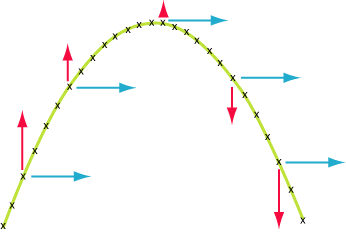Fun explodes with the solving of equations, making graphs along with understanding the real-life and practical use of this function. And one of its important characteristics is how to find the domain and range of a quadratic function or domain and range of a parabola in other words.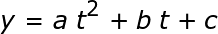In many places, you'll encounter a quadratic relation in physics with projectile motion.

Think that you're tossing a baseball straight up in the air. Let's try visualizing this with a height vs. time graph. Over time the ball goes up to a maximum height, and then back down to the starting height again when you catch it.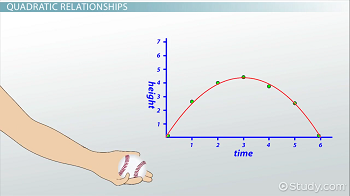We can see our graph creates an upside-down parabola, which is the sort of thing you might expect from a quadratic relation. Let us verify whether the relation between height and time is quadratic by looking at the vertical equation for projectile motion that deals with position and time: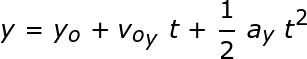Does it look familiar? Let's try rearranging the equation a bit: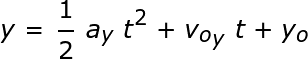Upon rearranging the terms, it comes out to be a quadratic function.So it is important for us to see the domain and range of a quadratic function to really understand the domain and range of a parabola.

## Domain and Range of a Parabola

The range of a function is the set of output values when all x-values within the domain are evaluated into the function, commonly referred to as the y-values. This means I want to seek out the domain first so as to explain the range.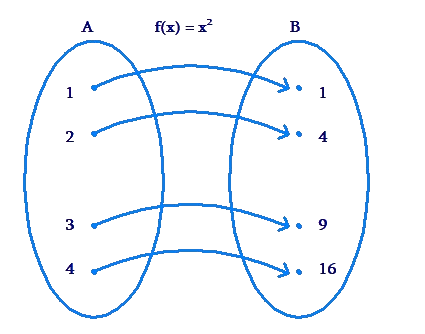Finding the range of a quadratic function may be a bit more tricky than finding the domain of a quadratic function. Sometimes you can use a graphing calculator to possess an accurate picture of the function. And, if you don’t want to use it, I encourage you to sketch a graph.

Either way, it's crucial that you simply have an honest idea of how the graph seems so as to properly describe the range of the function.

## How to find the domain and range of a quadratic function?

Let us have a step by step guidance on how to find the domain and range of a quadratic function. Here are some examples on domain and range of a parabola.

 Example 1

Find the domain and range of the linear function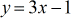### Solution

The equation given is clearly a purely linear equation which implies the coefficient of the square power is 0.

This makes the analysis much simpler.

### Domain of a quadratic function

Further, upon observation, there are not any x-values that will make the function not exist or invalid since no denominator or square root exists.

Thus, the domain is all x values.

The domain and range of such a function will come out to be: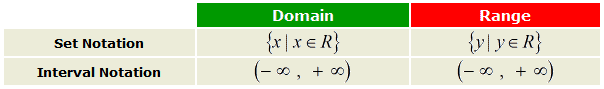### Range of a quadratic function

As the function is linear, the graph would come out to be a line.

The range is all y values.

It can certainly go as high or as low without any limits.

It is advisable to look at graphs for such observations: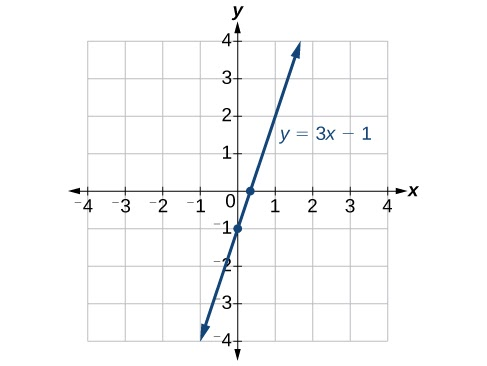Example 2

Find domain and range of quadratic function: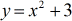### Domain of a quadratic function

Upon putting any values of x into the quadratic function, it remains valid and existing throughout. So, I can say that its domain is all x values.

But the range of a parabola is a little trickier.

It won’t be all possible values of y. Upon observing any parabola and trying to work out the domain and range of a parabola it is evident that it has a maxima or minima point at the tip of the curve.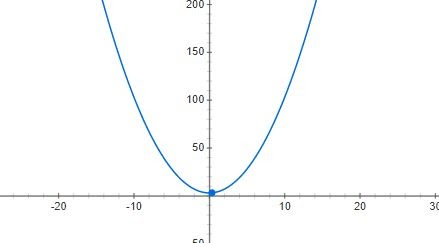### Range of a quadratic function

The graph of the parabola has a minima at y = 3 and it can have values higher than that. So such a characteristic leads to the range of quadratic function being: y ≥ 3.
Summary of domain and range of a parabola in tabular form: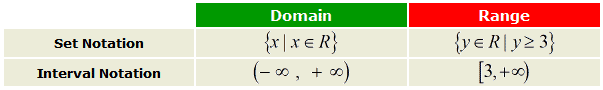Example 3

How to find the domain and range of a quadratic function: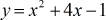### Domain of a quadratic function

This quadratic function will always have a domain of all x values. This was quite easy.

But now to find the range of the quadratic function:

### Range of a quadratic function

The parabola given is in the Standard Form, y = ax² + bx + c. So we should make our task easy and convert it into vertex form.

Vertex Form, y = a (x-h) ²+ k, where the vertex is (h,k)

Complete the Squares: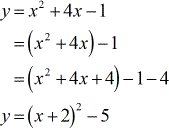Since the parabola opens upward, there must minima which would turn out to be the vertex. The coordinate of the minima is:This parabola evidently has a minimum value at y = −5, and can go up to positive infinity.

The range of quadratic function: y ≥ −5.

 Example 4

Find the domain and range of the quadratic function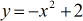### Solution

This is also a parabola since quadratic function.

### Domain of a quadratic function

Here, evaluating the domain of a parabola will include knowing that this will also have either a minimum or a maximum.

Since the coefficient of the x square term is negative, the parabola opens downward and therefore has a maximum (high point). The domain should be all x values because there are no values that when substituted to the function will yield “bad results”.

### Range of a quadratic function

Now, here one should be careful. People assume that parabolas will have a minimum and thus the vertex would be it.

Carefully observe the equation, the negative sign indicates that the parabola will actually face downward and the vertex will be the maxima of the function.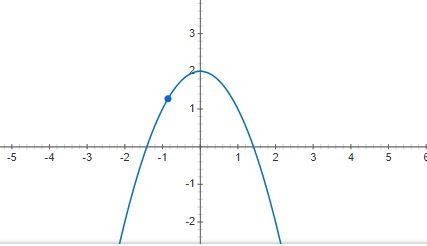Thus, the parabola has a maximum value at y = 2 and it can go down as low as it wants.
The range of parabola: y ≤ 2

The summary of the domain and range of a parabola is the following: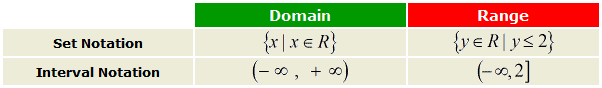## Summary

This lesson deals with equations involving quadratic functions which are parabolic. Quadratic equations are equations of the form y = ax2 + bx + c or y = a(x - h)2 + k.

The shape of the graph of a quadratic equation is a parabola. The first section of this chapter explains how to graph any quadratic equation of the form y = a(x - h)2 + k, and it shows how varying the constants a, h, and k stretches and shifts the graph of the parabola. Then we move onto actually finding the domain and range of a parabola using various examples.
Domain of a parabola or domain or a quadratic function can be easily observed by which values can be input.
Range of a parabola is a little more tricky and requires the help of a quadratic function graph.

Written by Gargi Shrivastava

## How to find the domain and range of a quadratic function?

Domain of a parabola or domain of a quadratic function would just be the set of values for which the function exists and is valid.

Finding the range of a quadratic function may be a bit more tricky than finding the domain of a quadratic function. Sometimes you can use a graphing calculator to possess an accurate picture of the function. And, if you don’t want to use it, I encourage you to sketch a graph.

Related Articles